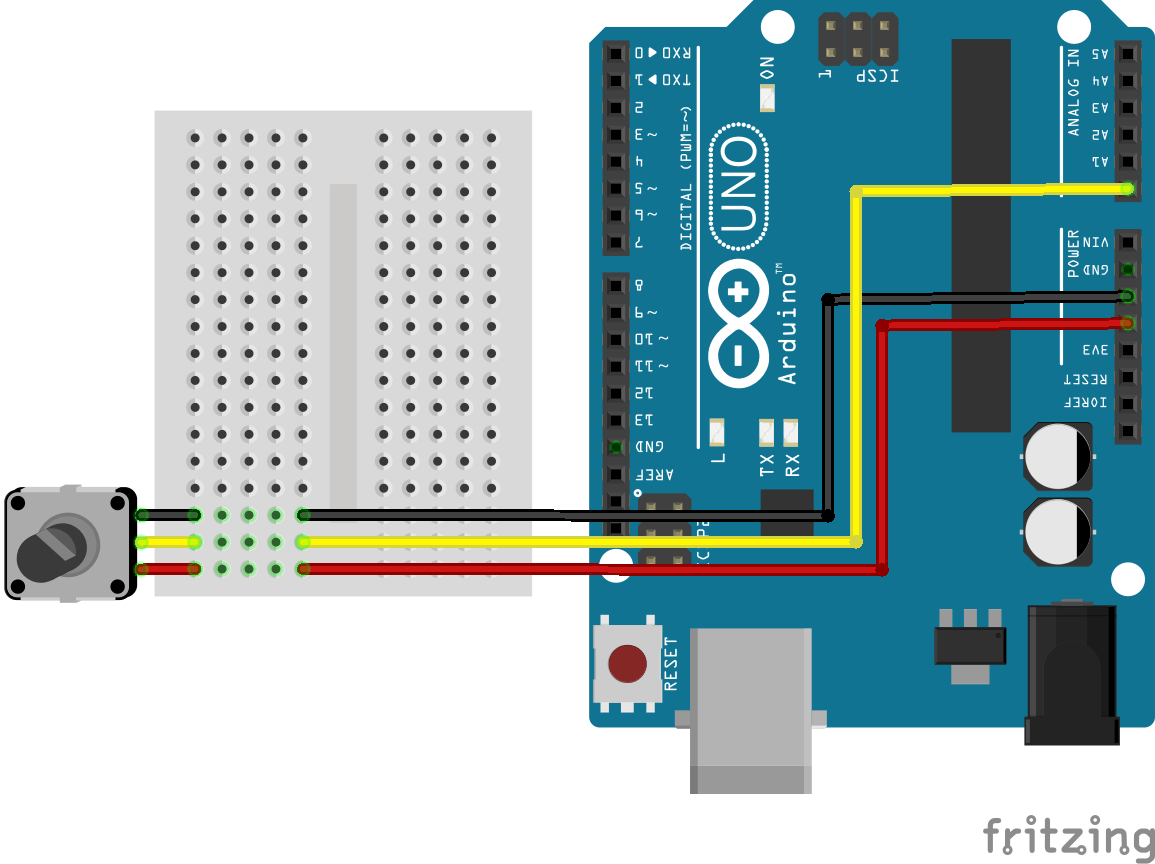Select Page
Tags: , , , ,
0
(0)
The potentiometer is a variable resistor which is used to vary the voltage at its terminals. It is used in several applications including set a value: adjust the brightness of a light, adjust the volume of a speaker, change the position of a servomotor, etc.

## Hardware

• Computer
• ArduinoUNO
• USB Cable
• Dupont Cable M/F x3

## Scheme

The potentiometer is a passive component. To measure a change in resistance, we send a current between the potentiometer terminals extreme and we can read the value of the resulting voltage divider created on the middle terminal.
The potentiometer must be connected as shown below.## Code

To display the physical value of the sensor, it is necessary to know the conversion rule is often linear type y = a * x + b. In order to have a clean and readable code, it is best to place the code in a sub function. We will create a function that is responsible for reading the sensor value and convert physical value.
To use the potentiometer object we use the following code:
```//Constants
#define NB_POT 1

//Parameters
const int potPin  = A0;

//Variables
int potVal  = 0;

void setup(){
//Init Serial USB
Serial.begin(9600);
Serial.println(F("Initialize System"));
//Init potentiometer
pinMode(potPin,INPUT);
}

void loop(){
}

Serial.print("Raw val : ");Serial.println(potVal);
Serial.print("Phys val : ");Serial.println(potRawToPhys(potVal));
delay(200);
}

float potRawToPhys(int raw){/* function potRawToPhys */
////Potentiometer conversion rule
float Vout = float(raw) * (5/float(1024));//Conversion analog to voltage
float phys = (R * (5 - Vout))/5;//Conversion voltage to resistance

return phys;
}

```

## Applications

• Control the brightness of an LED.

## Sources

Find other examples and tutorials in our Automatic code generator

Click on a star to rate it!

Average rating 0 / 5. Vote count: 0

No votes so far! Be the first to rate this post.

As you found this post useful...